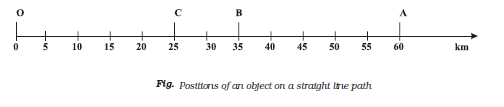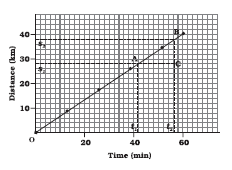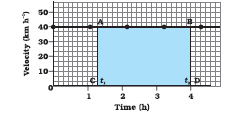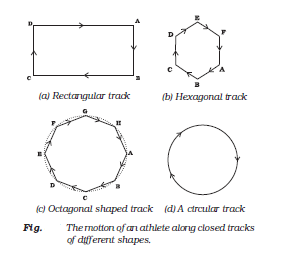# CBSE Class 9 Science Chapter 8 Motion Notes

Motion is referred to as a change of position and can be described in terms of distance moved or the displacement. The motion of an object could either be uniform or non-uniform depending on whether its velocity is changing or constant. Learn in detail through CBSE class 9 science chapter 8 motion notes right here at BYJU’S.

Chapter 8 Motion Notes:-

### Topics Covered In Chapter Motion

• Describing Motion
• Measuring the Rate of Motion
• Rate of Change of Velocity
• Graphical Representation of Motion
• Equations of Motion by Graphical Method
• Uniform Circular Motion

A particle is a point-like object having mass but miniscule size. The object’s position is the location of the object with respect to a particular reference point. Motion takes place when an object changes its position. When two objects are moving in a plane each has relative motion with respect to the second.

Speed- distance travelled in unit time. It is given by s = d/t where ‘d’ is the distance travelled and ‘t’ is the time taken. Its unit is metre/second

Distance – it is a measure of how far an object has moved. It is a scalar quantity.

Displacement – it covers the minimum distance between two points and is a vector quantity.

Motion Along A Straight Line

Motion along a straight line is the simplest type of motion. Consider an object moving along a straight path. The object starts its journey from O which is treated as its reference point. Let A, B and C indicate the position of the object at different instants. Initially, the object moves through C and B and reaches A. Then it moves back along the same path and reaches C through B.Different Types Of Speed

• Uniform speed – speed does not change
• Variable speed – riding a bike for 5km. Take off and increase speed, slowing down up hill, speed up downhill, stop sign
• Average speed = is given by total distance travelled/total time taken
• Instantaneous speed – it is the speed at any given point of time

Speed With Direction

The quantity that specifies both direction of motion along with speed is called velocity. Velocity is the speed of an object moving in a definite direction. The velocity of an object can be uniform or variable. It can be changed by changing the object’s speed, direction of motion or both. When an object is moving along a straight line at a variable speed, we can express the magnitude of its rate of motion in terms of average velocity. It is calculated in the same way as we calculate

average speed.

In case the velocity of the object is changing at a uniform rate, then average velocity is given by the arithmetic mean of initial velocity and final velocity for a given period of time. That is,

average velocity = (initial velocity + final velocity)/2

Rate Of Change Of Velocity

Acceleration is a measure of the change in the velocity of an object per unit time. That is,

acceleration = change in velocity/time taken

If the velocity of an object changes from an initial value u to the final value v in time t,

the acceleration a is,

a = (v – u)/t

This kind of motion is known as accelerated motion. The acceleration is taken to be positive if it is in the direction of velocity and negative when it is opposite to the direction of velocity. The SI unit of acceleration is m s-2.

If an object travels in a straight line and its velocity increases or decreases by equal amounts in equal intervals of time, then the acceleration of the object is said to be uniform. The motion of a freely falling body is an example of uniformly accelerated motion. On the other hand, an object can travel with non-uniform acceleration if its velocity changes at a non-uniform rate.

Graphical Representation Of Motion

To describe the motion of an object, we can use line graphs. In this case, line graphs show dependence of one physical quantity, such as distance or velocity, on another quantity, such as time

-> Distance – time graph

The change in the position of an object with time can be represented on the distance-time

graph using a convenient scale of choice. Here, time is taken along the x–axis and distance is taken along the y-axis. These graphs can be employed under different conditions where objects move with uniform speed, non-uniform speed, remain at rest etc.-> Velocity – time graphs

The variation in velocity with time for an object moving in a straight line can be represented by a velocity-time graph. In these graphs, time is represented along the x-axis and the velocity is represented along the y-axis.Velocity-time graph for a uniform motion of a car

Equations of Motion by Graphical Method

The motion of an object moving at uniform acceleration can be described with the help of the following equations, namely

v = u + at

s = ut + ½ at2

2as = v2 – u2

where u is initial velocity of the object, which moves with uniform acceleration a for time t, v is its final velocity and s is the distance it travelled in time t.

Uniform Circular Motion

If an object moves in a circular path with uniform speed, its motion is called uniform circular motion.NCERT Related Articles NCERT Solutions for Class 12 NCERT Solutions for Class 11 NCERT Solutions for Class 10 NCERT Solutions for Class 12 Maths NCERT Solutions for Class 11 Maths NCERT Solutions for Class 10 Maths NCERT Solutions for Class 12 Physics NCERT Solutions for Class 11 Physics NCERT Solutions for Class 10 Science NCERT Solutions for Class 12 Chemistry NCERT Solutions for Class 11 Chemistry NCERT Solutions for Class 6 NCERT Solutions for Class 12 Biology NCERT Solutions for Class 11 Biology NCERT Solutions for Class 6 Maths NCERT Solutions for Class 9 NCERT Solutions for Class 8 NCERT Solutions for Class 6 Science NCERT Solutions for Class 9 Maths NCERT Solutions for Class 8 Maths NCERT Solutions for Class 4 NCERT Solutions for Class 9 Science NCERT Solutions for Class 8 Science NCERT Solutions for Class 4 Maths NCERT Solutions for Class 5 NCERT Solutions for Class 7 NCERT Solutions for Class 7 Maths NCERT Solutions for Class 4 Science NCERT Solutions for Class 5 Maths NCERT Solutions for Class 7 Science NCERT Solutions NCERT Books NCERT Solutions for Class 5 Science